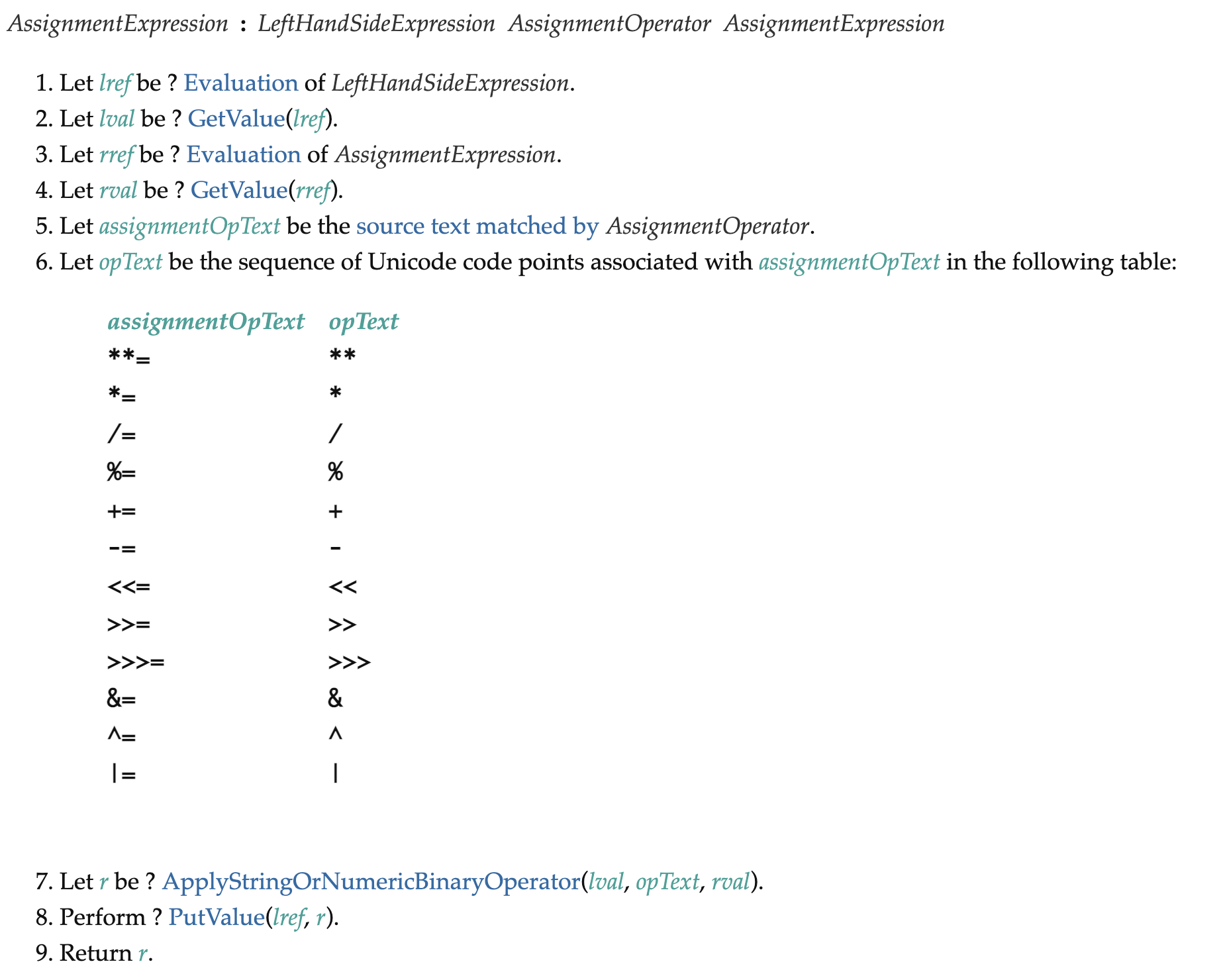# Race Conditions Using `+=` OperatorsThe JavaScript architecture for multi-tasking (the event loop, promises, and the async/await syntax sugar) is one of the most successful languages for reasoning about “concurrent” code.

Still, from time to time, JavaScript gives us some surprises. In particular, we’ve found surprising results when JavaScript is used in combination with assignment operators such as “+=.”

For example, see the following for a fairly common construct in parallel data processing:

```
const totalRecordsProcessed = 0;
await Promise.all(input.map(async (data) => {
totalRecordsProcessed += await processRecords(data);
});
```

To identify the problem with it, let’s simplify it to the following:

```
var total = 0;
await Promise.all([1,2,3].map(async (i) => total += await i));
console.log(total);
```

What do you expect to be the output of this last console.log?

But, if you’re like most of us, you answered “6,” which is wrong. Why? Because JavaScript, like other languages in the C family tree, defines assignment operators, such as “+=” and “*=”, as follows (excerpt from the ECMA-262 spec):In other words, this means that the value of the variable to be updated by the assignment operator is read before the expression on the right hand side is evaluated.

This is also visible in expressions with side-effects on the variable, such as x += x++, which will not increment x at all if it was initially zero.

This is somewhat counterintuitive because we expect

x += expr;

to be equivalent to

const y = expr;
x += y;

One way of thinking about this is to see the expression x += expr as short-hand for x = x + expr, and not for x = expr + x.

In order to prevent such errors, ESLint provides a rule—require-atomic-updates—which will detect occurrences of similar structures. We had previously disabled it in the distant past because it was overly aggressive. We are currently re-evaluating that decision.